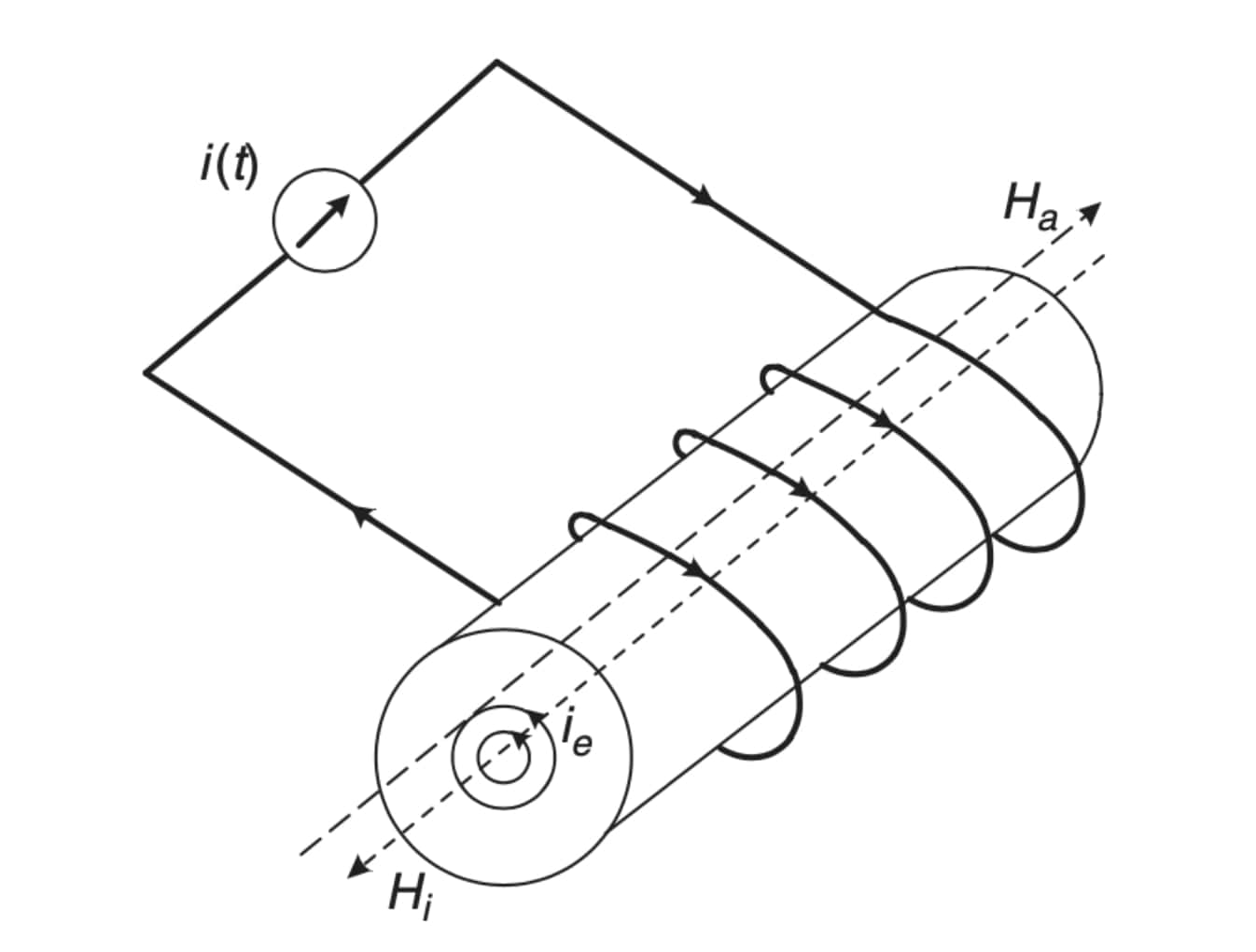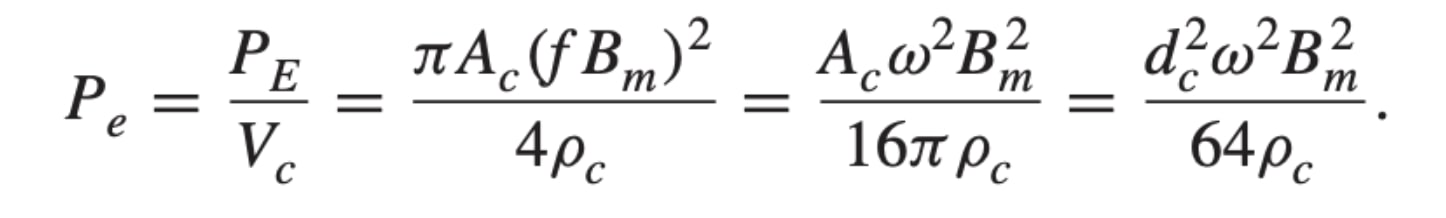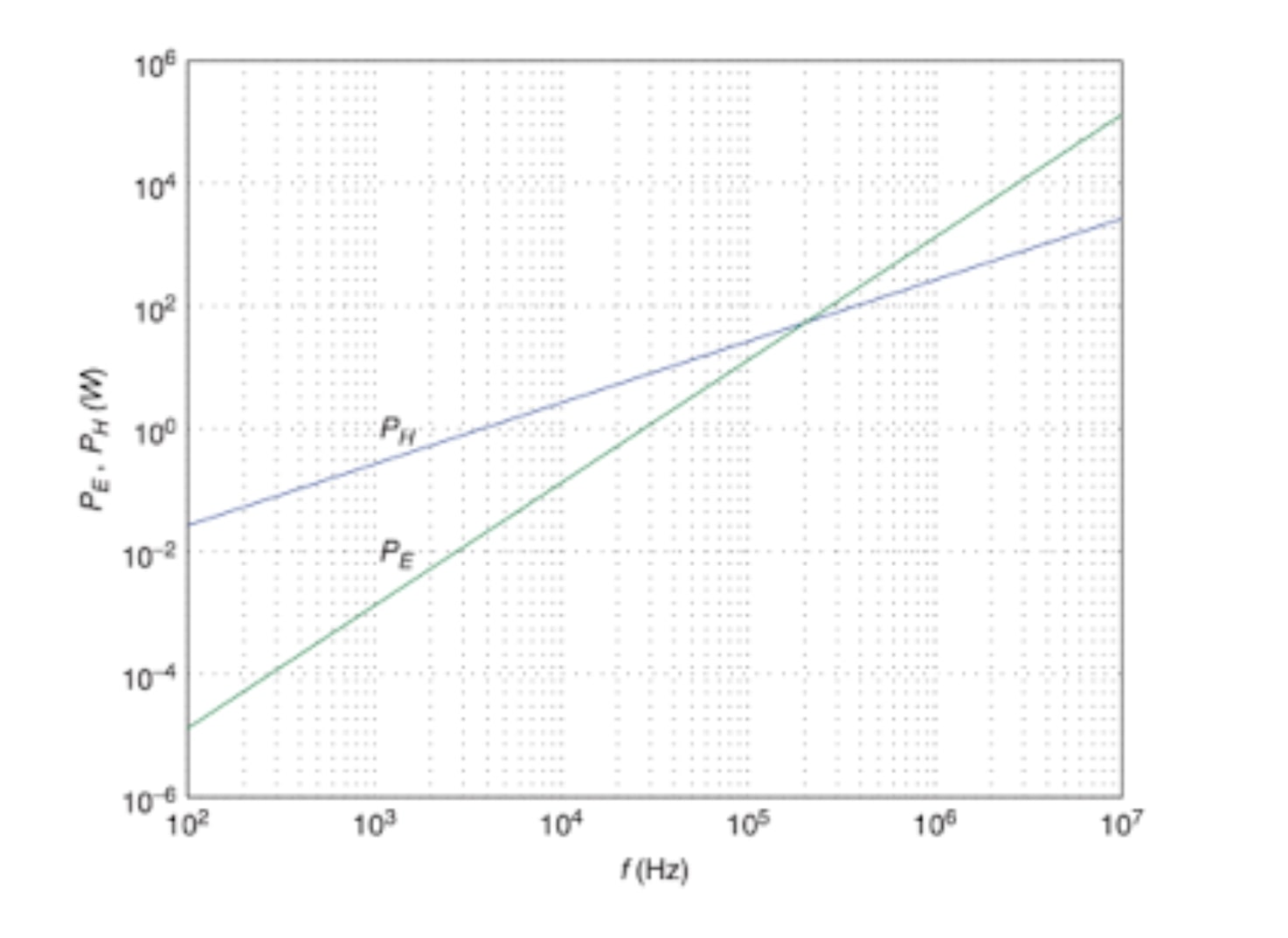# Eddy Current losses in Magnetic Components

The process of designing magnetics has been always full of physical effects which need to be considered during the design. One of my favorite effects (Favorite from a physical point of view) is the Eddy Currents. It's an effect like a kind of revenge for trying to use the relation of Electric currents for creating Magnetic fields and vice versa. In this brief article, I will describe the effect and calculate the losses with an example.

## How do the Eddy Currents appear?

If we consider an inductor, based on a wire around a magnetic core and we apply an AC current ia(t) through the wire, we will induce a magnetic field in the magnetic core Ha (t). Great, that was our initial goal. The intensity of this field will depend on the current and the physical parameters and dimensions of the parameters involved.## Here is when the revenge comes...

According to Lenz's law, the magnetic field Ha(t) will produce an electric current (Eddy Currents!) in the magnetic core, which depending on the magnetic core resistivity to electric currents would have a specific value.

This is the main reason for the low-frequency laminated cores. These cores were very good at conducting the electricity, therefore, the eddy currents are creating a lot of conduction losses in the core. However, in the ferrite materials, where the resistivity is much higher, it is less common to divide the core into different sections but in some cases is still needed.

But there is more. This Eddy Current will induce another magnetic field He(t) in opposite direction to the initial magnetic field Ha (t) reducing the total net value of the magnetic field created with ia (t). (What a traitor you will think..)

The losses density, considering a sinusoidal flux density are express with the following equation:As it is shown, the frequency and the Magnetic field increase the losses, while increasing the resistivity reduces them.

To show it in a real application, let's take an example (I take this example in ).

Consider an MnZn ferrite ungapped core with the following features:

• Ac= 80 mm2
• A mean MPL lc= 94 mm
• Relative permeability urc=2500
• Flux density Bs=320 mT at 20ºC
• Resistivity =1 Ohm x cm
• N = 10
• The voltage applied to the inductor VL= 10 sin( 2pi 100000)

Using the previous equation, the losses are Pe= 187 mW in this ferrite material, which represents a value that will increase with the frequency and shouldn´t be neglected.

Here in this graph are represented Eddy Current losses and Hysteresis losses in the function of the frequency.## This effect is the reason for the lamination of the low-frequency magnetic components.

Dividing the core into different laminates, the current only can be created in each independent one, with much less impact on the losses.

In the ferrite materials is not very common (because of the manufacturing costs), but in high-power applications, where the ferrite cores are huge, the Eddy Current is very common to use stacked cores instead of only one core. Reduce the thickness of the core, while keeping the same flux density are the main challenges for an optimal solution.

To conclude, Eddy Currents should be considered, especially in big magnetic components, not only due to the conduction losses in the core, but the resultant magnetic field created. In small transformers or inductors, the effect is not too relevant but could explain the lack of accuracy of your initial predictions.

### References

 Marian Kazimierczuk - High-Frequency Magnetic Components, Second Edition-John Wiley _ Sons, Ltd (2013)

Do you want the full version?